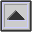Appendix B
UNITS OF MEASUREMENT

 Name Abbreviation Unit of Equivalent or definition atmosphere atm pressure 1 atm is normal atmospheric pressure 1 atm = 1.013 x 105 N/m2 = 1.013 bar = 14.7 lbs/in2 bar - pressure 1 bar = 106 dynes/cm2 = 106 µbar = 0.9869 atm bel - intensity level see decibel below cent - frequency ratio (interval) 1 cent corresponds to a frequency ratio of 21/1200 100 cents = 1 semitone 1200 cents = 1 octave cycles per second cps frequency 1 cps = 1 Hz (see hertz below) decibel dB intensity level 1 dB = 0.1 bel = log I1/I2 where I1 = 10.I2 dyne dyn force 1 dyn = 10-5 N = 1 gm cm/sec2 erg - energy 1 erg = 10-7 J = 1 gm cm2/sec2 gigahertz gHz frequency 1 gHz = 109 Hz = 103 mHz = 106 kHz hertz Hz frequency 1 Hz = 1 cycle/sec = 1 cps 1000 Hz = 1 kHz = 1 kc joule J energy 1 J = 107 erg = 1 kg m2/sec2 kilocycle kc 1000 cycles 1 kilocycle/sec = 1 kc = 1000 cps = 1 kHz kilohertz kHz frequency 1 kHz = 1000 Hz megacycle mc 106 cycles 1 megacycle/sec = 1 mc = 106 cps = 1 mHz megahertz mHz frequency 1 mHz = 106 Hz = 103 kHz mel - pitch 1000 Hz at 40 dB is defined as 1000 mels microbar µbar pressure 1 microbar = 10-6 bar = 1 dyne/cm2 Threshold of Hearing is 2 x 10-4 µbar microsecond µsec time 1 µsec = .001 ms = 10-6 sec mil - distance (thickness) 1 mil = .001 inch millisecond ms time 1 ms = .001 sec Newton N force 1 N = 105 dyn = 1 kg m/sec2 noy - noise Pascal Pa pressure 1 Pa = 1 N/m2 = 106 µPa phon - loudness sabin - absorption 1 sabin is equivalent to 1 squre foot of a surface absorbing all incident energy sone - loudness 1 sone = 40 phons watt w energy flow or power 1 watt = 1 joule/sec

* The terms kilocycle and megacycle, just as the basic term cycle, are often used loosely meaning frequency units. Proper frequency specification would be cycles/sec, kilocycles/sec, megacycles/sec. Partly due to this ambiguity, ther terms hertz, kilohertz and megahertz have been introduced as standard units of frequency.

home